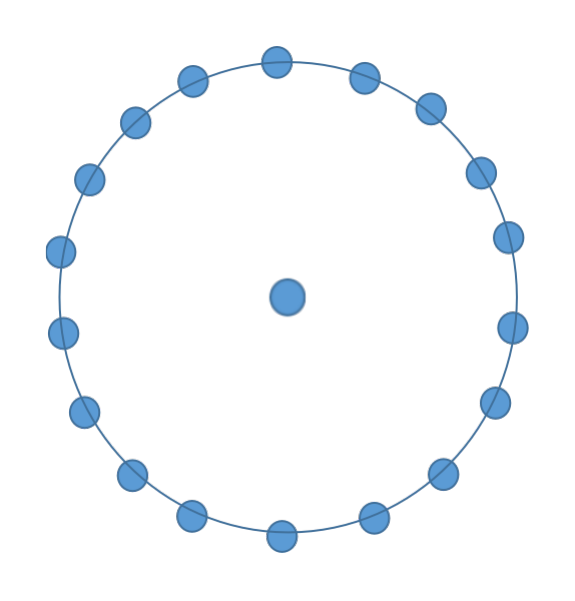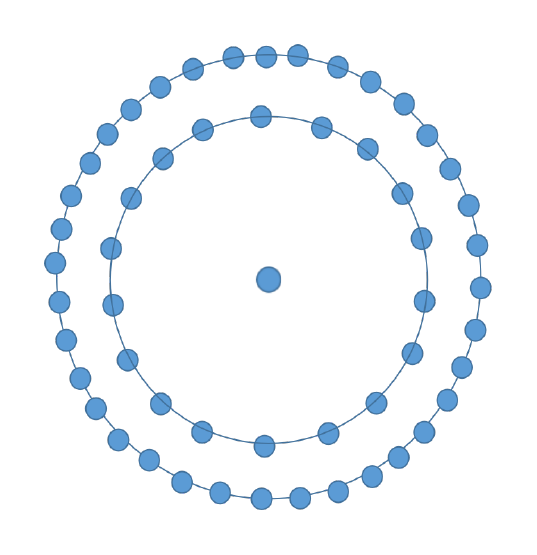The problem includes to print the N-th centered octadecagonal number, where N will be given as an input.

A centered octadecagonal number is a type of figurative number which is represented as a dot in the centre surrounded by the successive layers of the octadecagon. An octadecagon is a polygon with 18 sides in it. The successive layers of the octadecagon are the first layer will be 18-sided polygon, the next will be 36-sided polygon and so on.

The numbers can be better explained with the help of figures.The first number is represented as a dot in the centre therefore the first centered octadecagonal number is 1.The second number in the sequence of centered octadecagonal number is represented as a dot in the centre surrounded by an octadecagon. Thus the number will be 19.The next number in the sequence of centered octadecagonal number is represented as a dot in the centre surrounded by the two successive layers of the octadecagon i.e. 18-sided polygon and a 36-sided polygon. Thus, the number will be 55.

Following the same pattern in the next figures, we can calculate the next numbers in the sequence of centered octadecagonal numbers. The first few centered octadecagonal numbers are 1, 19, 55, 109, 181, 271, 379…….

In this problem, N will be provided as an input and we need to print the value of the centered octadecagonal number corresponding to the value of N.

Example −

INPUT : N=4
OUTPUT : 109


Explanation − Here we are given N=4. The value of the centered octadecagonal number corresponding to N i.e. 4th number is 109 which is represented as a dot in the centre surrounded by 3 successive layers of the octadecagon.

INPUT : N=7
OUTPUT : 379


Explanation − The 7th centered octadecagonal number is 379 represented as a dot in the centre surrounded by 6 successive layers of an octadecagon i.e. 18, 36, 54, 72, 90 and 108.

Let’s try to come up with an algorithm to solve the above algorithm.

## Algorithm

The idea behind the algorithm to solve this problem is by observing the pattern in every centered octahedral number. As we saw in the figurative representation of the numbers, every number is represented as a dot in the centre surrounded by the successive layers of an octadecagon.

Any centered octadecagonal number can be found by 1 + sum of (N-1) successive layers of an octadecagon. The successive layers of an octadecagon are 18, 36, 54, 72…… which is forming an AP with first term, a=18 and common difference, d=18.

The N-th centered octadecagonal number can be represented in the form of mathematical relation as −

$$\mathrm{(centered \: octadecagonal)_N=1+sum \:of(N−1)successive \: layers \: of \: an \: octadecagon}$$

The sum of first n terms of an AP can be found by,

$$\mathrm{S_n=\frac{n}{2}(2*a+(n−1)d)}$$

To find the sum of first (N-1) terms of successive layers of an octadecagon, we can use the above formula.

$$\mathrm{(centered \: octadecagonal)_N=\frac{(N −1)}{2}(2*a+(N−2)d)+1}$$

Replacing a=18 and d=18 as the first term of AP is 18 and the common difference is also 18.

$$\mathrm{(centered \: octadecagonal)_N=\frac{(N −1)}{2}(2*18+(N − 2)*18)+1}$$

$$\mathrm{(centered \: octadecagonal)_N=\frac{18*(N − 1)}{2}(2+N − 2+1)}$$

$$\mathrm{=\frac{18*N*(N − 1)}{2}+1}$$

$$\mathrm{9N(N −1)+1}$$

Every N-th centered octadecagonal number can be found with the above expression i.e. 9𝑁(𝑁 − 1) + 1 where N>0. We will use this expression in our approach to solve the problem efficiently.

## Approach

Below are the steps to follow to implement the algorithm in our approach −

• We will make a function in order to calculate the value of the N-th centred octadecagonal number for any value of N greater than 0.

• Make a variable to store the value of the N-th number. We must ensure that the variable is of long long data type to store the number for the larger values of N.

• Use the formula, 9N(N − 1) + 1 to get the N-th centered octadecagonal number and store it in the variable created.

• Return the number and print it which will be our required output.

The C++ code for the approach −

### Example

//C++ code to print the N-th centered octadecagonal number
#include <bits/stdc++.h>

using namespace std;

//function to calculate the N-th centered octadecagonal number
long long Nth_num(int N){
//store the N-th number in n
long long n = 9*N*(N-1) + 1; //using the general expression of

// N-th centered octadecagonal number i.e. 9N(N-1)+1
return n; //return the N-th number
}
int main(){
int N;
N=9;

//calling the function
cout<<"The N-th centered octadecagonal number is "<<Nth_num(N)<<endl;
N=17;
cout<<"The N-th centered octadecagonal number is "<<Nth_num(N)<<endl;
return 0;
}


### Output

The N-th centered octadecagonal number is 649
The N-th centered octadecagonal number is 2449


Time Complexity − O(1) , because we took constant time to perform the operation.

Space Complexity − O(1) , as no extra space is taken.

## Conclusion

The concepts related to centered octadecagonal numbers and their representation in the form of figures were discussed in the article. We derived a formula for the N-th centered octadecagonal number which we used in our approach to solve the problem efficiently within constant time and space in C++.